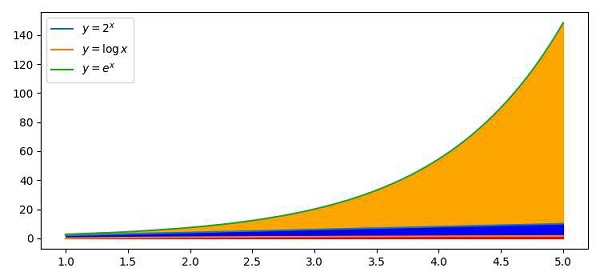# How can I create a stacked line graph with matplotlib?

To create a stacked lines graph with Python, we can take the following Steps −

• Create x, y, y1 and y2 points using numpy.

• Plot the lines using numpy with the above data (Step 1) and labels mentioned.

• Fill the color between curve y=e^x and y=0, using the fill_between() method.

• Fill the color between curve y=2x and y=0, using the fill_between() method.

• Fill the color between curve y=log(x) and y=0, using fill_between() method.

• Place the curve text using the legend() method.

• To display the figure, use the show() method.

## Example

import matplotlib.pyplot as plt
import numpy as np
plt.rcParams["figure.figsize"] = [7.50, 3.50]
plt.rcParams["figure.autolayout"] = True
x = np.linspace(1, 5, 100)
y = x * 2
y1 = np.log(x)
y2 = np.exp(x)

plt.plot(x, y, label=r'$y=2^x$')
plt.plot(x, y1, label=r'$y=\log{x}$')
plt.plot(x, y2, label=r'$y=e^x$')
plt.fill_between(x, y2, 0, color='orange')
plt.fill_between(x, y, 0, color='blue')
plt.fill_between(x, y1, 0, color='red')
plt.legend()
plt.show()

## Output